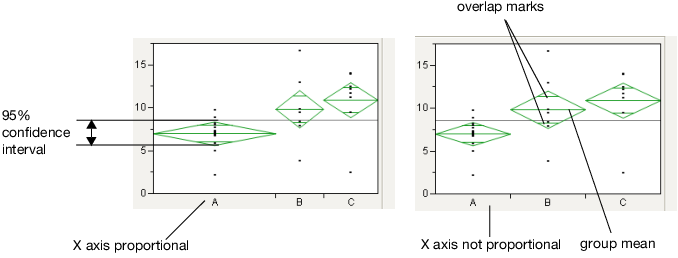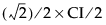Basic Analysis > Oneway Analysis > Means/Anova and Means/Anova/Pooled t > Mean Diamonds and X-Axis Proportional

Publication date: 06/16/2020

## Mean Diamonds and X-Axis Proportional

A mean diamond illustrates a sample mean and confidence interval.

Figure 6.8 Examples of Mean Diamonds and X-Axis Proportional OptionsNote the following observations:

The top and bottom of each diamond represent the (1-alpha)x100 confidence interval for each group. The confidence interval computation assumes that the variances are equal across observations. Therefore, the height of the diamond is proportional to the reciprocal of the square root of the number of observations in the group.

If the X-Axis proportional option is selected, the horizontal extent of each group along the horizontal axis (the horizontal size of the diamond) is proportional to the sample size for each level of the X variable. Therefore, the narrower diamonds are usually taller, because fewer data points results in a wider confidence interval.

The mean line across the middle of each diamond represents the group mean.

Overlap marks appear as lines above and below the group mean. For groups with equal sample sizes, overlapping marks indicate that the two group means are not significantly different at the given confidence level. Overlap marks are computed as group mean ±. Overlap marks in one diamond that are closer to the mean of another diamond than that diamond’s overlap marks indicate that those two groups are not different at the given confidence level.

The mean diamonds automatically appear when you select the Means/Anova/Pooled t or Means/Anova option from the platform menu. However, you can show or hide them at any time by selecting Display Options > Mean Diamonds from the red triangle menu.